Courses

# Fluid Mechanics: Assignment Part - 1 Notes | EduRev

## IIT JAM : Fluid Mechanics: Assignment Part - 1 Notes | EduRev

The document Fluid Mechanics: Assignment Part - 1 Notes | EduRev is a part of the IIT JAM Course Mechanics & General Properties of Matter.
All you need of IIT JAM at this link: IIT JAM

Q.1. A U-tube of uniform cross-sectional area, open to the atmosphere, is partially filled with mercury. Water is then poured into both arms. If the equilibrium configuration of the tube is as shown in figure, with h2 = 1.00 cm , determine the value of h1.

Let h be the height of the water column added to the right side of the U-tube. Then when equilibrium is reached, the situation is as shown in the sketch at right. Now consider two points, A and B shown in the sketch, at the level of the water-mercury interface. By Pascal’s Principle, the absolute pressure at B is the same as that at A . But,
PA = P0 + ρwgh + ρHggh2 and PB = P0 + ρwg(h1 + h + h2)
Thus, from PA = PB, ρwh1 + ρwh2 + ρwh + ρHgh2 or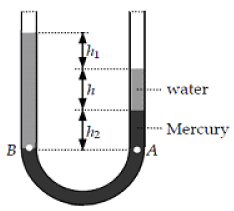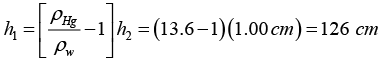Q.2. Mercury is poured into a U-tube as in figure. The left arm of the tube has cross-sectional area A1 of 10.0 cmand the right arm has a cross-sectional area A2 of 5.00 cm2. One hundred grams of water are then poured into the right arm as in figure
(a). Determine the length of the water column in the right arm of the U-tube.
(b) Given that the density of mercury is 13.6 g/cm3, what distance h does the mercury rise in the left arm?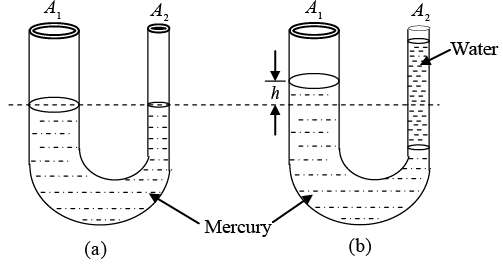(a) Using the definition of density, we have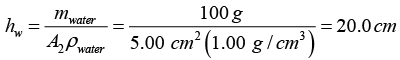Sketch (b) at the right represents the situation after the water is added. A volume (A2h2) of mercury has been displaced by water in the right tube. The additional volume mercury now in the left tube is A1h. Since the total volume of mercury has not changed,
A2h2 = A1h or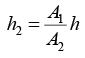...(i)
At the level of the mercury-water interface in the right tube, we may write the absolute pressure as: P = P+ ρwaterghw
The pressure at this same level in the left tube is given by
P = P0 + ρHgg(h + h2) = P0 + ρwaterghw
which, using equation (i) above, reduces to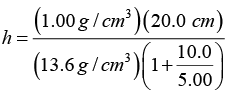= 0.490 cm above the original level.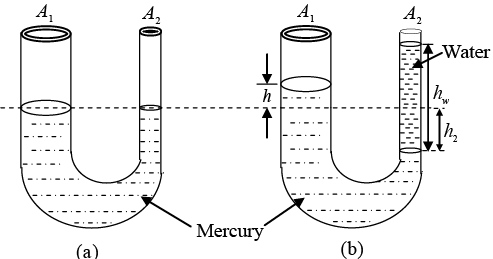Q.3. A spherical aluminum ball of mass 1.26 kg contains an empty spherical cavity that is concentric with the ball. The ball just barely floats in water. Calculate
(a) the outer radius of the ball and
(b) the radius of the cavity.

(a) The weight of the ball must be equal to the buoyant force of the water:
1.26 kgg =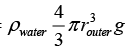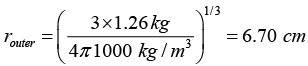(b) The mass of the ball is determined by the density of aluminum: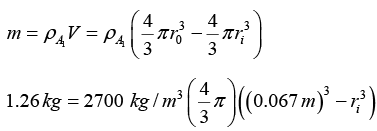1.11 x 10-4m3 = 3.01 x 10-4 m3 - ri3
ri = (1.89 10-4m3)1/3 = 5.74 cm

Q.4. A cube of wood having an edge dimension of 20.0 cm and a density of 650 kg/m3 floats on water.
(a) What is the distance from the horizontal top surface of the cube to the water level?
(b) How much lead weight has to be placed on top of the cube so that its top is just level with the water?

(a) According to Archimedes,
H = ρwaterVwater g =(1.00 g/cm3)[20.0 x 20.0 x 20.0 - h)] g
But B = Weight of block = mg = ρwoodVwoodg = (0.650 g/cm3)(20.0 cm)2g
0.650(20.0)3g = 1.00(20.0)(20.0)(20.0 -h)g
20.0 - h = 20.0(0.650) so h = 20.0(1-0.650) = 7.00 cm
(b) B = Fg + Mg where M = mass of lead
1.00(20.0)3 g = 0.650(20.0)3 g + Mg
M = (1.00 - 0.650)(20.0)3 = 0.350(20.0)3 = 2800 g = 2.80 kg

Q.5. A cylindrical object of diameter 10 cm, height 20 cm and density 8000 kg/m3 is supported by a vertical spring and is half dipped in water.
(a) Find the elongation of the spring in equilibrium condition.
(b) If the object is slightly depressed and released, find the time period of resulting oscillations of the object. (Spring constant = 500 N /m)

Volume of the cylinder (V) = πrx H
V = 3.14 x 52  x 20 = 1571 cm3
Mass of the cylindrical object (M) = ρV
M = (8000 kg/m3)(1571 x 10-6 m3) = 12.57 kg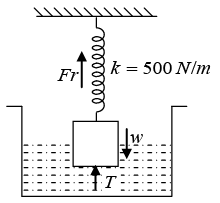(a) When object is half dipped, then the volume displaced in water is Vd = Half volume of cylinder = 785.4 cm3 = 785.4 x 10-6 m3
In equilibrium conditions; W = Fr + T ⇒ Fr = W - T
Weight of object W = rρVg
W = (8000 kg/m3)(1571 x 10-6 m3) (10 ms-2) = 125.7 N
Force of buoyancy, T = ρwVdg = (1000 kg/m3)(785.4 x 10-6 m3)(10 ms-2)
T = 7.85N
Then F= W - T = 125.7 - 7.85 = 117.85

Also,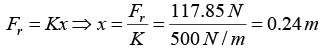(b) The time period of oscillation is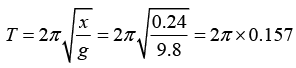T = 0.98 sec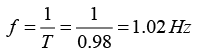Q.6. A block of mass 1kg and density 0.8g/cm3 is held stationary with the help of a string as shown in figure. The tank is accelerating vertically upwards with an acceleration a = 1.0m/s2. Find
(a) the tension in the string
(b) if the string is now cut find the acceleration of block
(Take g = 10m/s3 and density of water 103 kg/m3)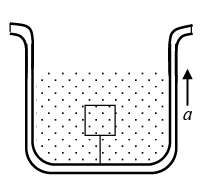(a) Free body diagram of the block is shown in figure.
In the figure, F = Up thrust force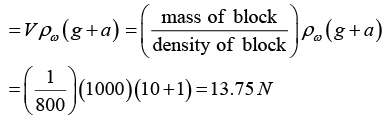W = mg = 10N
Equation of motion of the block is,
F -T -W = mα
∴ 13.75 - T - 10 = 1x1
∴ T = 2.75N
(b) When the string is cut, T = 0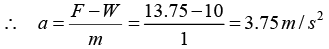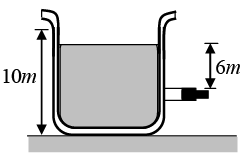Q.7. A cubical block of wood of edges 3 cm float in water. The lower surface of the cube just touches the free end of a vertical spring fixed at the bottom of the pot. Find the maximum weight that can be put on the block without wetting it.
(density of wood = 800 kg/m3 and spring constant = 50 N/m).

Suppose the maximum weight that can be put without wetting it is W . The block in this case is completely immersed in the water. The volume of the displaced water is (Vd)
Vd = Volume of block = α= (3 cm)3
Vd = 27 x 10-6 m3
The force of byoyancy T = (mass of displaced liquid)g
T = Vd ρωg = (27 x 10-6 m3) (1000 kg/m3)(9.8 m)
T = 0.27N
Let us calculate the portion of wood dipped in water
Height inside water =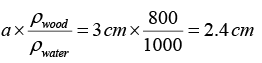∴ Height outside water = 3 cm - 2.4 cm = 0.6 cm
The spring is compressed by 0.6 cm and hence the upward force exerted by the spring is
Fr = Kx = (50 N/m)(0.6 x 10-2m) = 0.3
Fr = 0.3N
The force of buoyancy and the spring force taken together balance the weight of the block plus the weight W put on the block.
The weight of block W' = ρwood x V x g
W' = (800 kg/m3)(27 x 10-6 m3) (9.8 ms-2)
W' = 0.21N
Thus upward force = downward force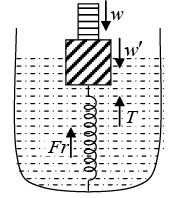T + Fr = W + W'
0.27 + 0.3 = W + 0.21 ⇒ W = 0.36N

Q.8. A small block of wood, of density 0.4 x 103 kg/m3, is submerged in water at a depth of 2.9m. Find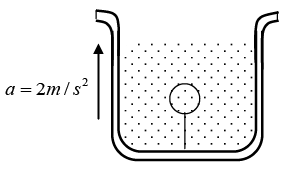(a) the acceleration of the block toward the surface when the block is released and
(b) the time for the block to reach surface. Ignore viscosity.

(a) upthrust-weight/mass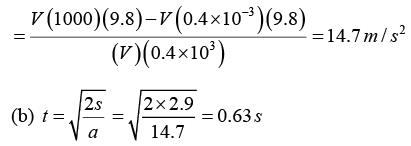Q.9. A wooden rod weighing 25N is mounted on a hinge below the free surface of water as shown. The rod is 3m long and uniform in cross section and the support is 1.6m below the free surface. At what angle a will it come to rest when allowed to drop from a vertical position. The cross-section of the rod is 9.5x10-4 m2 in area. Density of water is 100kg/m3. Assume buoyancy to act at center of immersion. g = 9.8m/s2. Also find the reaction on the hinge in this position.

Let G be the mid-point of AB and E the mid point of AC (i.e. the centre of

buoyancy)
AC = 1.6 cosec α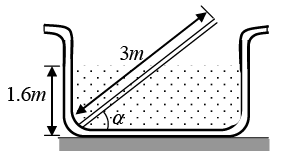Volume of AC = (1.6x9.5x10-4) cosec α
Weight of water displaced by AC
= (1.6 x 9.5 x 10-4 x 103 x 9.8) cosec α = 14.896 cosec α
Hence, the buoyant force is = 14.896 cosec α acting vertically upwards at E. While the weight of the rod is 25 N acting vertically downwards at G. Taking moments about A,
(14.896 cosec α) (AE cos α) = (25) (AG cos α)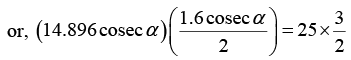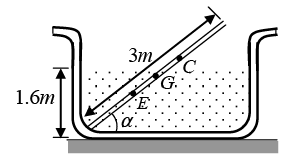or, sin2 α = 0.32 ∴ sin α = 0.56
or, α = 34.30
Further, let F be the reaction at hinge in vertically downward direction. Then, considering the translatory equilibrium of rod in vertical direction we have,
F + weight of the rod = up thrust
F = up thrust - weight of the rod
= 14.896 cosec (34.30) - 25 = 26.6 - 25
F = 1.6N (downwards)

Q.10. With what terminal velocity will an air bubble 0.8mm in diameter rise in a liquid of viscosity 0.15 N -s/m2 and specific gravity 0.9? Density of air is 1.293 kg/m3.

The terminal velocity of the bubble is given by,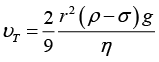Here, r = 0.4 x 10-3 m,  σ = 0.9 x 103 kg/m3  ρ = 1.293kg/m3 η = 0.15, N - s/mand g = 9.8m/s2 Substituting the values, we have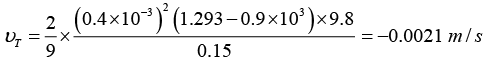or vr = 0.21 cm/s

Q.11. A cylindrical object of outer diameter 10 cm, height 20 cm and density 8000 kg/m3 is supported by a vertical spring and is half dipped in water as shown in figure.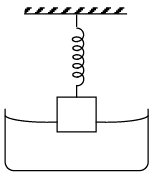(a) Find the elongation of the spring in equilibrium condition.
(b) If the object is slightly depressed and released, the time period of resulting oscillations of the object. The spring constant = 500 N/m.

(a) Let x be the elongation of spring from mean position.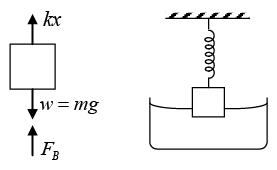kx + FB = mg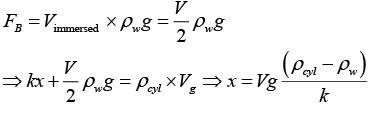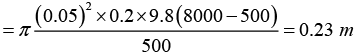(b) Cylinder is depressed by distance y. Additional forces are F'B due to immersed part and spring force ky.
Restoring force = ky + F'B = ky+ Ay ρwg
A is cross section area of cylinder.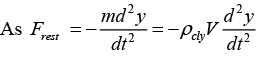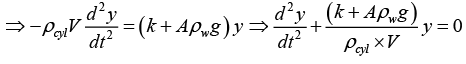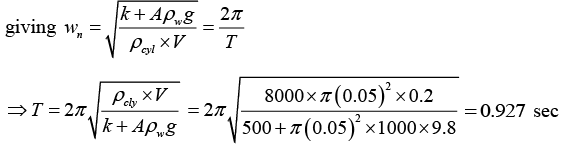Q.12. Water flows through a tube shown in figure. The areas of cross-section at A and B are 1cmand 0.5 cm2 respectively. The height difference between A and B is 5 cm . If the speed of water at A is 10 cm/sec, find (a) the speed at B and (b) the difference in pressures at A and B.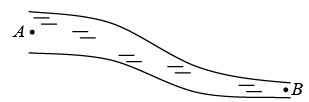Equation of continuity A1V1 = A2V2
Given A1 = 1cm2, A2 = 0.5 cm2,  V1 = 10cm/sec
∴ V2 = A1/A2 V1 = 1/0.5 x 10 = 20cm/sec
(b) According to Bernauli equation:
P1 + 1/2 ρV12 ρgh1 = P2 + 1/2 ρV22 + ρgh2
P2 - P1 = ρg(h1 - h2) + 1/2 ρ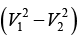= 1000 x 10 x 5 x 10-2 + 1000/2 (10 + 20) x 10-2 x (10 - 20) x 10-2
= 500 - 15 = 485 Pα

Q.13. Water level is maintained in a cylindrical vessel upto a fixed height H. The vessel is kept on a horizontal plane. At what height above the bottom should a hole be made in the vessel so that the water stream coming out of the hole strikes the horizontal plane at the greatest distance from the vessel?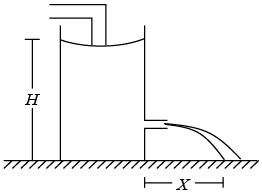Let hole is at height h from base Applying Bernoulli’s equation between (1) and (2), taking datum at base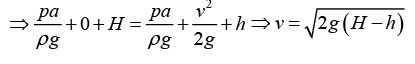with volume V water takes time t to strike the ground, h = 0 + 1/2 gt2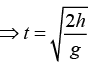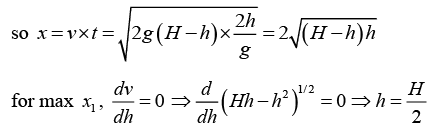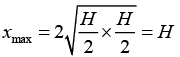Q.14. A cube of ice floats partly in water and partly in K. oil. Find the ratio of the volume of ice immersed in water to that in K. oil. Specific gravity of K.oil is 0.8 and that of ice is 0.9.

Let Side of cube = α
As weight of cube = total buoyancy force
W = FBoil + FBwater
⇒ α3 x ρrig = (α - x) x α2 x ρoilg + xα2 ρw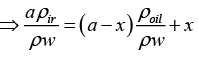α x 0.9 = (α - x) x 0.8 + x
⇒ 0.9α = 0.8α - 0.8x + x ⇒ x = 0.1α/0.2 = 0.5α
Cube is half immersed in water and half in oil.

Q.15. Water leaks out from an open tank through a hole of area 2 mm2 in the bottom. Suppose water is filled up to a height of 80 cm and the area of cross-section of the tank is 0.4 m2. The pressure at the open surface and at the hole are equal to the atmospheric pressure. Neglect the small velocity of the water near the open surface in the tank.
(a) Find the initial speed of water coming out of the hole.
(b) Find the speed of water coming out when half of water has leaked out.
(c) Find the volume of water leaked out during a time interval dt after the height remained is h. Thus find the decrease in height dh in terms of h and dt.
(d) From the result of part (c) find the time required for half of the water to leak out.

(a)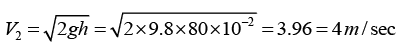(b) (c) (i) Q = A2V(cm3/sec)
Volume leaked in time dt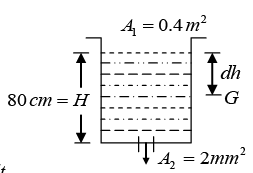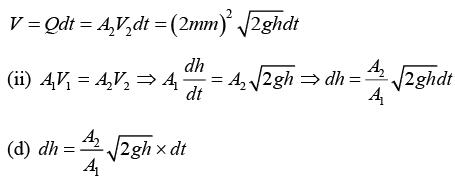Offer running on EduRev: Apply code STAYHOME200 to get INR 200 off on our premium plan EduRev Infinity!

## Mechanics & General Properties of Matter

2 videos|23 docs|25 tests

,

,

,

,

,

,

,

,

,

,

,

,

,

,

,

,

,

,

,

,

,

;